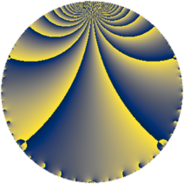# Properties

 Label 52.2.hLevel $52$ Weight $2$ Character orbit 52.h Rep. character $\chi_{52}(17,\cdot)$ Character field $\Q(\zeta_{6})$ Dimension $2$ Newform subspaces $1$ Sturm bound $14$ Trace bound $0$

# Related objects

## Defining parameters

 Level: $$N$$ $$=$$ $$52 = 2^{2} \cdot 13$$ Weight: $$k$$ $$=$$ $$2$$ Character orbit: $$[\chi]$$ $$=$$ 52.h (of order $$6$$ and degree $$2$$) Character conductor: $$\operatorname{cond}(\chi)$$ $$=$$ $$13$$ Character field: $$\Q(\zeta_{6})$$ Newform subspaces: $$1$$ Sturm bound: $$14$$ Trace bound: $$0$$

## Dimensions

The following table gives the dimensions of various subspaces of $$M_{2}(52, [\chi])$$.

Total New Old
Modular forms 20 2 18
Cusp forms 8 2 6
Eisenstein series 12 0 12

## Trace form

 $$2 q + q^{3} - 3 q^{7} + 2 q^{9} + O(q^{10})$$ $$2 q + q^{3} - 3 q^{7} + 2 q^{9} - 9 q^{11} - 2 q^{13} - 3 q^{17} + 9 q^{19} - 3 q^{23} + 10 q^{25} + 10 q^{27} + 9 q^{29} - 9 q^{33} - 9 q^{37} + 5 q^{39} - 9 q^{41} - 5 q^{43} - 4 q^{49} - 6 q^{51} - 12 q^{53} + 9 q^{59} + 5 q^{61} - 6 q^{63} + 3 q^{67} + 3 q^{69} + 9 q^{71} + 5 q^{75} + 18 q^{77} + 8 q^{79} - q^{81} - 9 q^{87} + 27 q^{89} - 3 q^{91} + 6 q^{93} - 21 q^{97} + O(q^{100})$$

## Decomposition of $$S_{2}^{\mathrm{new}}(52, [\chi])$$ into newform subspaces

Label Dim $A$ Field CM Traces $q$-expansion
$a_{2}$ $a_{3}$ $a_{5}$ $a_{7}$
52.2.h.a $2$ $0.415$ $$\Q(\sqrt{-3})$$ None $$0$$ $$1$$ $$0$$ $$-3$$ $$q+(1-\zeta_{6})q^{3}+(-2+\zeta_{6})q^{7}+2\zeta_{6}q^{9}+\cdots$$

## Decomposition of $$S_{2}^{\mathrm{old}}(52, [\chi])$$ into lower level spaces

$$S_{2}^{\mathrm{old}}(52, [\chi]) \cong$$ $$S_{2}^{\mathrm{new}}(13, [\chi])$$$$^{\oplus 3}$$